# Statistical vector generation for multivariate normal distributions – I – multivariate and bi-variate normal distributions from CAEs

This post requires Javascript to display formulas!

# Convolutional Autoencoders and multivariate normal distributions

Experiments as with my own on convolutional Autoencoders [CAE] show: A CAE maps a training set of human face images (e.g. CelebA) onto an approximate multivariate vector distribution in the CAE’s latent space Z. Each image corresponds to a point (z-point) and a corresponding vector (z-vector) in the CAE’s multidimensional latent space. More precisely the results of numerical experiments showed:

The multidimensional density function which describes the inner dense core of the z-point distribution (containing more than 80% of all points) was (aside of normalization factors) equivalent to the density function of a multivariate normal distribution [MND] for the respective z-vectors in an Euclidean coordinate system.

For results of my numerical CAE-experiments see
Autoencoders and latent space fragmentation – X – a method to create suitable latent vectors for the generation of human face images
and related previous posts in this blog. After the removal of some outliers beyond a high sigma-level (≥ 3) of the original distribution the remaining core distribution fulfilled conditions of standard tests for multivariate normal distributions like the Shapiro-Wilk test or the Henze-Zirkler test.

After a normalization with an appropriate factor the continuous density functions controlling the multivariate vector distribution can be interpreted as a probability density function [p.d.f.]. The components vj (j=1, 2,…, n) of the vectors to the z-points are regarded as logically separate, but not uncorrelated variables. For each of these variables a component specific value distribution Vj is given. All these marginal distributions contribute to a random vector distribution V, in our case with the properties of a MND:

$\boldsymbol{V} \: = \: \left( \, V_1, \, V_2, \, ….\, V_n\, \right) \: \sim \: \boldsymbol{\mathcal{N_n}} \, \left( \, \boldsymbol{\mu} , \, \boldsymbol{\Sigma} \, \right), \\ \quad \mbox{with} \: \boldsymbol{\mathcal{N_n}} \: \mbox{symbolizing a MND in an n-dimensional space}$

μ is a vector with all mean values μj of the Vj component distributions as its components. Σ abbreviates the covariance matrix relating the distributions Vj with one another.

The point distribution of a CAE’s MND forms a complex rotated multidimensional ellipsoid with its center somewhere off the origin in the latent space. The latent space itself typically has many dimensions. In the case of my numerical experiments the number of dimension was n ≥ 256. The number of sample vectors used were between 80,000 and 200,000 – enough data to approximate the vector distribution by a continuous density function. The densities for the Vj-distributions formed a smooth Gaussian function (for a reasonable sampling interval). But note: One has to be careful: The fact that the Vj have a Gaussian form is not a sufficient condition for a MND. (See the next post.) But if a MND is given all Vj have a Gaussian form.

# Generative use of MNDs in multidimensional latent spaces of high dimensionality

When we want to use a CAE as a generative tool we need to solve a problem: We must create statistical vectors which point into the (multidimensional) volume of our point distribution in the latent space of the encoding algorithm. Only such vectors provide useful information to the Decoder of the CAE. A full multivariate normal distribution and hyperplanes of its multidimensional density function are difficult to analyze and to control when developing a proper numerical algorithm. Therefore I want to reduce the problem of vector generation to a sequence of viewable and controllable 2-dimensional problems. How can this be achieved?

A central property of a multivariate normal distribution helps: Any sub-selection of m vector-component distributions forms a multivariate normal distribution, too (see below). For m=2 and for vector components indexed by (j,k) with respective distributions Vj, Vk we get a so called “bivariate normal distribution[BND]:

$V_{jk} \: \sim \: \mathcal{N}_2\left(\, (\mu_j, \mu_k), (\,\sigma_j, \sigma_k \,) \, \right)$

A MND has n*(n-1)/2 such subordinate BNDs. The 2-dim density function of a bivariate normal distribution

$g_{jk}\,\left( \, v_j, \, v_k\, \right) \: = \: g_{jk}\,\left( \, v_j, \, v_j, \, \mu_j, \, \mu_k, \, \sigma_j, \sigma_k, \, \sigma_{jk}, … \right)$

for vector component values vj, vk defines a point density of the sample data in the (j,k)-coordinate plane of the Euclidean coordinate system in which the MND is described. The density function of the marginal distributions Vj showed the typical Gaussian forms of a univariate normal distribution.

The density function of a BND has some interesting mathematical properties. Among other things: The hyperplanes of constant density of a BND’s density function form ellipses. This is illustrated by the following plots showing such contour lines for selected pairs (Vj, Vk) of a real point-distribution in a 256-dimensional latent space. The point distribution was created by a CAE in its latent space for the CelebA dataset.

Contour lines for selected (j,k)-pairs. The thick lines stem from theory and calculated correlation coefficients of the univariate distributions.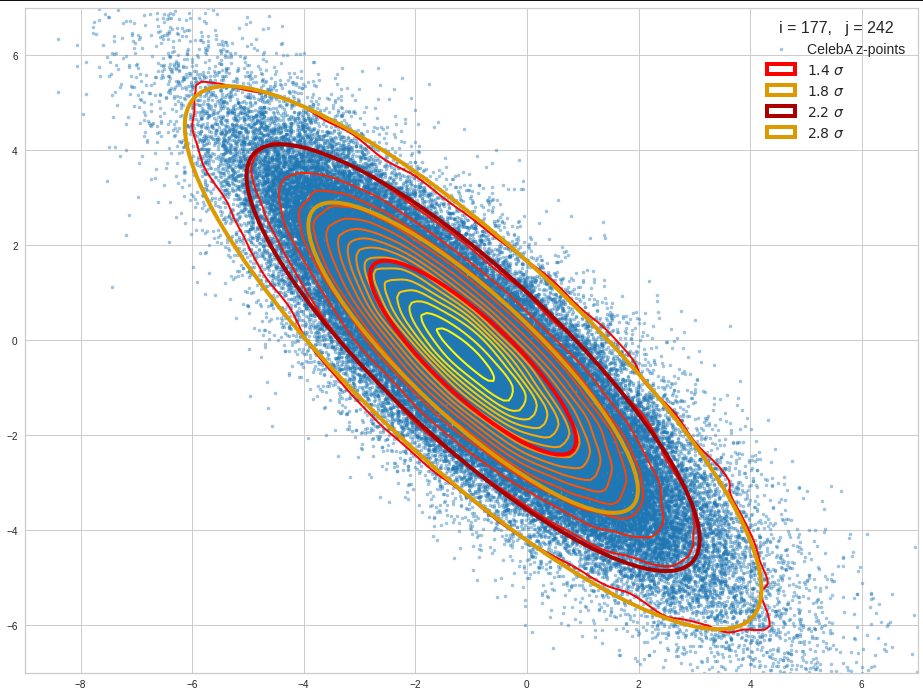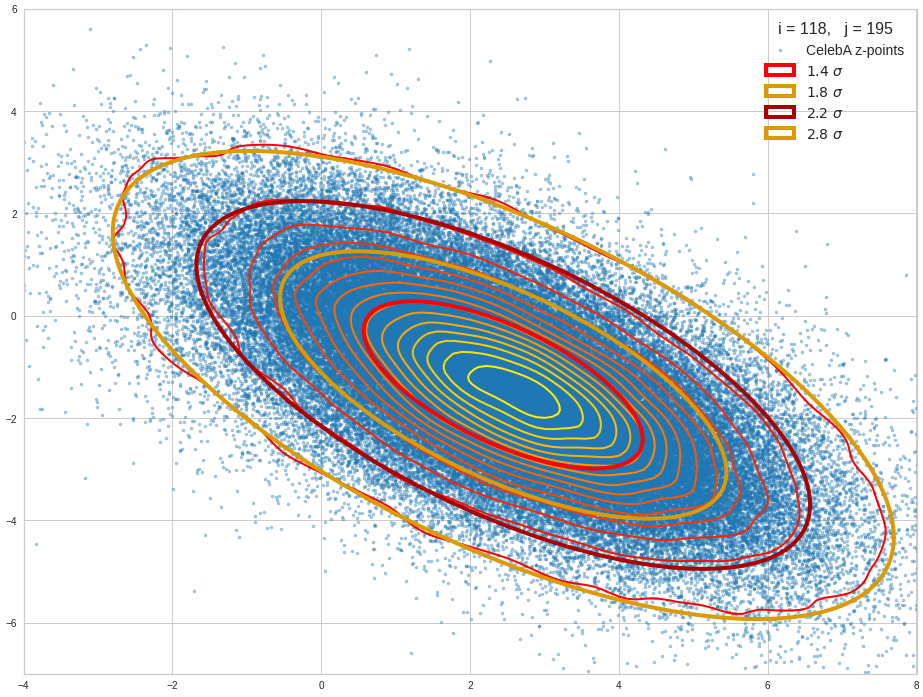The next plot shows the contours of selected vector-component pairs after a PCA-transformation of the full MND. (Main ellipse axes are now aligned with the axes of the PCA-coordinate system):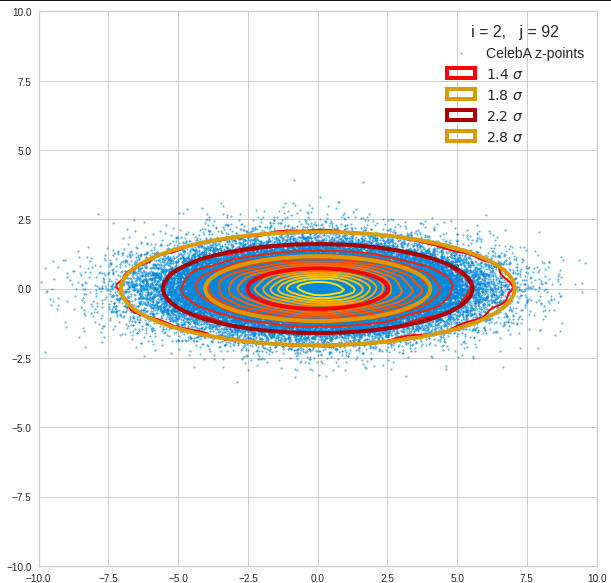These ellipses with axes along the coordinate axes are relatively easy to handle. They can be used for vector creation. But they require a full PCA transformation of the MND-distribution, a PCA-analysis for complexity reduction and an application of the inverse PCA-transformation. The plot below shows the point-density compared to a 2.2-σ-confidence ellipses. The orange points are the results of a proper statistical numerical vector generation algorithm based on a PCA-transformation of the MND.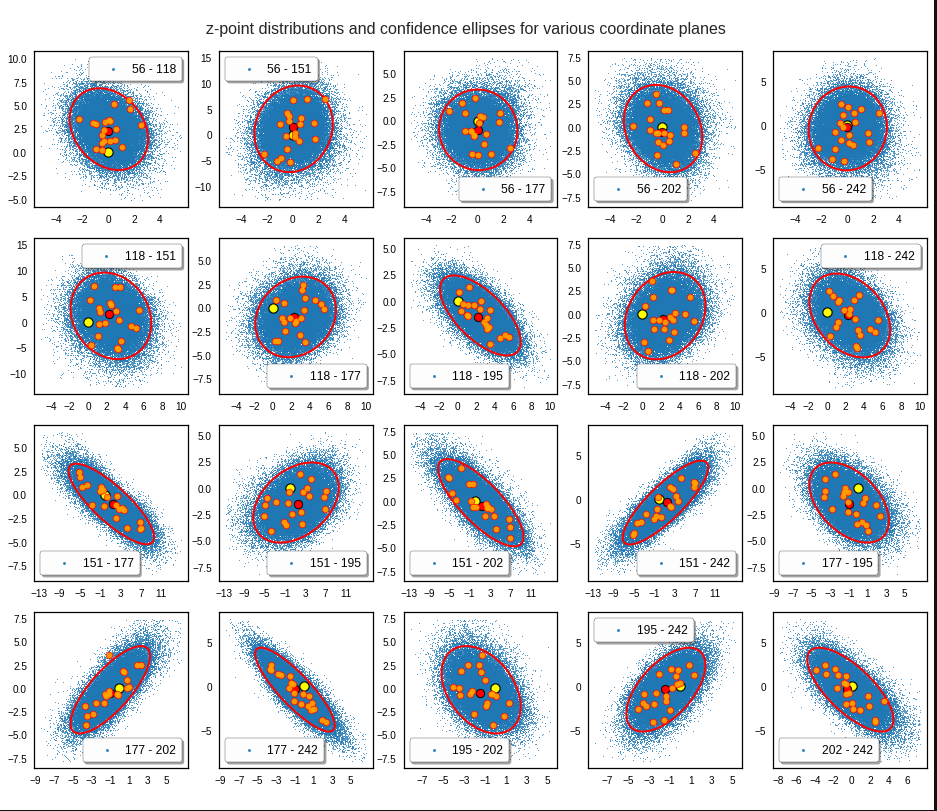See my post quoted above for the application of a PCA-transformation of the multidimensional MND for vector creation.

However, we get the impression that we could also use these rotated ellipses in projections of the MND onto coordinate planes of the original latent space system directly to impose limiting conditions on the component values of statistical vectors pointing to an inner regions of the MND. Of course, a generated statistical vector must then comply with the conditions of all such ellipses. This requires an analysis and combined use of the ellipses of all of the subordinate BNDs of a the original MND during an iterative or successive definition of the values for the vector components.

# Objective of this post series

In my last post about CAEs (see the link given above) I have explicitly asked the question whether one can avoid performing a full PCA-transformation of the MND when creating statistical vectors pointing to a defined inner region of a MND.

The objective of this post series is to prove the answer: Yes, we can. And we will use the BNDs resulting from projections of the original MND onto coordinate planes. We will in particular explore the n*(n-1)/2 the properties of the BNDs’ confidence ellipses. As said: These ellipses are rotated against the coordinate system’s axes. We will have to deal with this in detail. We will also use properties of their 1-dimensional marginal distributions (projections onto the coordinate axes, i.e. the Vj.)

In addition we need to prepare a variety of formulas before we are able to define numerical procedure for the vector generation without a full PCA-transformation of the MND with around 100,000 vectors. Some of the derived formulas will also allow for a deeper insight into how the multiple BNDs of a MND are related between each other and with confidence hypersurfaces of the MND.

Ellipses in general lead to equations governed by quadratic or fourth power polynomials. We will in addition use some elementary correlation formula from statistics and for some exercises a simple optimization via derivatives. The series can be regarded as an excursion into some of the math which governs bivariate distributions resulting from a MND.

As MNDs may also be the result of other generative Machine Learning algorithms in respective latent spaces, the whole approach to statistical vector generation for such cases should be of general interest. Note also that the so called “central limit theorem” almost guarantees the appearance of MNDs in many multivariate datasets with sufficiently large samples and value dependencies on many singular observations.

Distributions of a variety of variables may result in a MND if the variables themselves depend on many individual observables with limited covariance values of their distributions. In particular pairwise linearly correlated Gaussian density distributions individual variables (seen as vector components) may constitute a MND if the conditional probabilities fulfill some rules. We will see a glimpse of this in 2 dimensions when we analyze integrals over Gaussians in the bivariate normal case.

# Other approaches to statistical vector generation?

Well, we could try to reconstruct the multidimensional density function of the MND. This is a challenge which appears in some problems of pure statistics, but also in experimental physics. See e.g. a paper of Rafey Anwar, Madeline Hamilton, Pavel M. Nadolsky (2019, Department of Physics, Southern Methodist University, Dallas; https://arxiv.org/pdf/1901.05511.pdf). Then we would have to find the elements of the (inverse) covariance matrix or – equivalently – the elements of a multidimensional rotation matrix. But the most efficient algorithms to get the matrix coefficients again work with projections onto coordinate planes. I prefer to use properties of the ellipses of the bivariate distributions directly.

Note that using the multidimensional density function of the MND directly is not of much help if we want to keep the vectors’ end points within a defined multidimensional inner region of the distribution. E.g.: You want to limit the vectors to some confidence region of the MND, i.e. to keep them inside a certain multidimensional ellipsoidal contour hyper-surface. The BND-ellipses in the coordinate planes reflect the multidimensional ellipsoidally shaped contour hyperplanes of the full distribution. Actually, when we vertically project a multidimensional hyperplane onto a coordinate plane then the outer 2-dim border line coincides with a contour ellipse of the respective BND. (This is due to properties of a MND. We will come back to this in a future post.) The problem of proper limiting individual vector component values thus again is best solved by analyzing properties of the BNDs.

# Steps, methods, mathematical level

As a first step I will, for the sake of completeness, write down the formula for a multivariate normal distribution, discuss a bit its mathematical construction from uncorrelated univariate normal distribution. I will also list up some basic properties of a MND (without proof!). These properties will justify our approach to create statistical vectors pointing into a defined inner region of the MND by investigating projected contour ellipses of all subordinate BNDs. As a special aspect I want to make it at least plausible, why the projected contour ellipses define infinitesimal regions of the same relative probability level as their multidimensional counterparts – namely the multidimensional ellipsoidal hypersurfaces which were projected onto coordinate planes.

Then as a first productive step I want to motivate the specific mathematical form of the probability density function [p.d.f] of a bivariate normal distribution. In contrast to many of the math papers I have read on the topic I want to use a symmetry argument to derive the basic form of the p.d.f. I will point out an important, but plausible assumption about conditional distributions. An analogous assumption on the multidimensional level is central for the properties of a MND.

As the distributions Vj and Vk can be correlated we then want to understand the impact of the correlation coefficients on the parameters of the 2-dimensional density function. To achieve this I will again derive the density function by using our previous central assumption and some simple relations between the expectation values of the constituting two univariate distributions in the linear correlation regime. This concludes the part of the series where we get familiar with BNDs.

Furthermore we are interested in features and consequences of the 2-dimensional density functions. The contour lines of the 2-dim density function are ellipses – rotated by some specific angle. I will look at a formal mathematical process to construct such ellipses – in particular confidence ellipses. I will refer to the results Carsten Schelp has provided in an Internet article on this topic.

His construction process starts with a basic ellipse, which I will call base correlation ellipse [BCE]. The length of the axes of this ellipse are eigenvalues of the covariance matrix of the standardized marginal distributions constituting the BND. The main axes of this elementary ellipse are in addition aligned with the two selected axes of a basic Euclidean coordinate system in which the bivariate distribution is defined. The length of the BCE’s main axes can be shown to depend on the correlation coefficient for the two vector component distributions Vj and Vk. This coefficient also appears in the precision matrix of the BND. Points on the base correlation ellipse can be mapped with two steps of an affine transformation onto points on the real contour ellipses, in particular to points of the confidence ellipses.

The whole construction process is not only of immense help when designing visualization programs for the contour ellipses of our distribution with many (around 100,000) individual vectors. The process itself gives us some direct geometrical insights. It also helps to avoid finding a numerical solution of the usual eigenvector-problems when answering some specific questions about the rotated contour ellipses. Normally we solve an eigenvalue-problem for the covariance matrix of the multi- or the many subordinate bi-variate distributions to get precise information about contour ellipses. This corresponds to a transformation of the distributions to a new coordinate system whose axes are aligned to the main axes of the ellipses. Numerically this transformation is directly related to a PCA transformation of the vector distributions. However, such a PCA-transformation can be costly in terms of CPU time.

Instead, we only need a numerical determination of all the mutual the correlation coefficients of the univariate marginal distributions of the MND. Then the eigenvalue problem on the BND-level is already analytically solved. We therefore neither perform a full numerical PCA analysis of the MND and multidimensional rotation of the vectors of around 100,000 samples. Nor do we analyze explained variance ratios to determine the most important PCA components for dimensionality reduction. We neither need to perform a numerical PCA analysis of the BNDs.

Most important: Our problem of vector generation is formulated in the original latent space coordinate system and it gets a direct solution there. The nice thing is that Schelp’s construction mechanism reduces the math to the solution of quadratic polynomial equations for the BNDs. The solutions of those equations, which are required for our ultimate purpose of vector generation, can be stated in an explicit form.

Therefore, the math in this series will mostly remain on high school level (at least at a level given when I was young). Actually, it was fun to dive back into exercises reminding me of school 50 years ago. I hope the interested reader has some fun, too.

# Solutions to some particular problems with respect to the confidence ellipses of the MND’s BNDs

In particular we will solve the following problems:

• Problem 1: The two points on the BCE-ellipse with the same vj-value are not mapped onto points with the same vj-value on the confidence ellipse. We therefore derive the coordinates of points on the BCE-ellipse that give us one and the same vj-value on the real confidence ellipse.
• Problem 2: Plots for a real MND vector distribution indicate that all (n-1) confidence ellipses of distribution pairs of a common Vj with other marginal distributions Vk (for the same confidence level and with k ≠ j) have a common tangent parallel to one coordinate axis. We will derive the value of a maximum v_j-value for all ellipses of (j,k)-pairs of vector component distributions. We will prove that it is identical for all k. This will define the common interval of allowed vj-component-values for a bunch of confidence ellipses for all (Vj, Vk)-pairs with a common Vj.
• Problem 3: The BCE-ellipses for a common j-, but different k-values depend on different values for the correlation coefficients ρj,k of Vj with its various Vk counterparts. Therefore we need a formula that relates a point on the BCE-ellipse leading to a concrete v_j-value of the mapped point on the confidence ellipse of a particular (Vj, Vk)-pair to respective points on other BCE-ellipses of different (Vj, Vm)-pair with the same resulting v_j-value on their confidence ellipses. I will derive such a formula. It will help us to apply multiple conditions onto the vector component values.
• Problem 4: As a supplemental exercise we will derive a mathematical expression for the size of the main axes and the rotation angle of the ellipses. We should, of course, get values that are identical to results of the eigenwert-problem for the correlation matrix (describing a PCA coordinate transformation). This gives us additional confidence in Schelps’ approach.

In the end we can use our results to define a numerical algorithm for the direct creation of vectors pointing to a defined inner region of the multivariate normal distribution. As said, this algorithms does not require a costly PCA transformations of the full MND or many, namely n*(n-1)/2 such PCA-transformations of its BNDs.

I intend to visualize all results with the help of a concrete example of a multivariate example distribution created by a CAE for the CelebA dataset. The plots will use Schelp’s construction algorithm for the confidence ellipses extensively.

# Conclusion and outlook

Convolutional Autoencoders create approximate multivariate normal distributions [MND] for certain input data (with Gaussian pattern properties) in their latent space. MNDs appear in other contexts of machine learning and statistics, too. For evaluation and generative purposes one may need statistical vectors with end points inside a defined multidimensional hypersurface corresponding to a certain confidence level and a certain constant density value of the MND’s density function. These hypersurfaces are multidimensional ellipsoids.

We have the hope that we can use mathematical properties of the MND’s subordinate bivariate normal distributions [BNDs] to create statistical vectors with end points inside the multidimensional confidence ellipsoids of a MND. Typically such an ellipsoid resides off the origin of the latent space’s coordinate system and the ellipsoid’s main axes are rotated against the axes of the coordinate system. We intend to base the confining conditions on the components of the aspired statistical vectors on correlation coefficients of the marginal vector component distributions. Our numerical algorithm should avoid a full PCA-transformation of the multidimensional vector distribution.

In the next post of this series I give a formula for the density function of a multivariate normal distribution. In addition I will list up some basic properties which justify the vector generation approach via bivariate normal distributions.

# Autoencoders and latent space fragmentation – X – a method to create suitable latent vectors for the generation of human face images

My present post series explores options to use a standard convolutional Autoencoder [AE] for the creation of images with human faces. The face generation should based on random input to the AE’s Decoder. On our quest for a suitable method we have meanwhile learned a lot about other aspects of Autoencoders, vector distributions in multi-dimensional latent spaces and generative methods for our special case:

• Methods to create statistical latent vectors [z-vectors] as input for the AE’s Decoder must be chosen carefully. Among other things: It is difficult to create a bunch of random vectors which cover wider areas in the vastness of a multidimensional space. So the z-vector creation must be adjusted to specific requirements.
• After having been trained with CelebA images a convolutional AE fills a limited and coherent region in the latent space with z-points for the training images. This latent space region appears to be critical for successful image creation: Statistically generated z-vectors should point to this region. The core of the z-point distribution gets filled relatively densely.
• A convolutional AE maps human face images onto an approximate multivariate normal distribution. This gives the inner core of the z-point distribution the structure of a multidimensional ellipsoid. The projections of this ellipsoid onto 2-dimensional coordinate planes show characteristic nested elliptic contour lines.
• As the main axes of these ellipses were inclined with different angle towards the axes of chosen coordinate planes we concluded that linear correlations mark average dependencies between the z-vector components. Limiting conditions imposed by these correlations must also be fulfilled by z-vectors used as the Decoder’s input.

See previous posts in this series for more details. In particular, the last 2 posts

Autoencoders and latent space fragmentation – IX – PCA transformation of the z-point distribution for CelebA

Autoencoders and latent space fragmentation – VIII – approximation of the latent vector distribution by a multivariate normal distribution and ellipses

have shown that the density distribution for the z-points really exhibits elliptic contour lines in the original coordinate system of the latent space and (!) in the target coordinate system of a PCA transformation.

In this post we use our gathered knowledge: I present a first simple method to generate z-vectors which point to the latent space region filled by z-points for CelebA images. These z-vectors will fulfill the general and limiting elliptic conditions for their components.

# Decomposing the full problem of latent vector generation into a sequence of 2-dimensional problems

The nice thing about multivariate Gaussian distributions with linear correlations between the vector components is the following: We can reduce the problem of choosing proper component values to a series of 2-dimensional restrictions. Firstly we can use characteristic properties of the Gaussian distribution for each component. And secondly we can use confidence ellipses in 2-dimensional coordinate planes to restrict the component values to allowed intervals.

Ellipses are most easy to handle when their axes are aligned with the axes of the coordinate system in which we describe them. So, let us assume that we know an affine transformation T to a new coordinate system which also has orthogonal axes and supports the following special transformation properties for a multivariate normal density distribution:

1. T maps nested elliptic contour lines of the multidimensional density distribution and in particular confidence ellipses for component pairs in the original coordinate system to nested elliptic contours and confidence ellipses in the new coordinate system.
2. Taligns the centers of the transformed ellipses with the origin of the new coordinate system.
3. T aligns the main axes of the mapped ellipses with the axes of the new coordinate system.
4. T is reversible.

How could we then use the transformed data for vector-creation?

In the new coordinate system, a contour ellipse in a chosen coordinate plane for the axes-indices (i, j) may have main diameters of size

d1 = 2 * a    and    d2 = 2 * b.

We then can first select a random v_i value to fall into a range [-a * fact, a * fact].

fact * a    <    v_i    <    fact * a

With fact being a proper factor. This factor defines a confidence level in the new coordinate system. With the value of v_i fixed and b being the half-diameter in the orthogonal direction the correlation condition for the z-point distribution says that the v_j value must fall into an interval [-c, c] defined by:

-c    <    v_j    <    c,
with c = b * fact * sqrt(1 – x**2 / (fact * a)**2)

But within these limits we can again choose the v_j-value freely. Below I use a simple random-function for a constant probability density to pick a value.

However: It would not be enough to restrict the coordinates to the conditions of just one ellipse! The components of the created vectors must in parallel fulfill elliptic conditions for all of the possible pairs of vector-components. I.e. we may need to adapt the v_j values gained from the analysis of a fist 2D-ellipse to further conditions of other ellipses and component pairs. This can be achieved by an iteration. For z_dim = 256 this involves a total of 32640 checks and possible value-adaptions to each and all of the allowed value ranges.

In addition: The order by which the component-pairs and their conditions are investigated must be randomized to get real statistical vector distributions.

Eventually the resulting vector components must be re-transformed into the original coordinate system of the latent space.

The ellipse for the “core’s boundary” in the original coordinate system will be defined by the chosen confidence level of the ellipsoidal normal distribution. We saw already that a confidence level of σ = 2.0 defines the transition to outer regions of the z-point density distribution quite well.

This all sounds manageable by relative simple Python programs. But: Do we know a proper transformation T? Yes, we do: A PCA-transformation of the z-point density distribution has all the properties discussed above.

# Using half maximum values after a PCA transformation of the z-point distribution

The last post proved that a PCA transformation maps ellipses onto ellipses for component pairs in the transformed PCA coordinate system. The advantage of the ellipses there is that their main axes are on average well aligned with the orthogonal PCA coordinate axes. Gaussians for the number density distribution per component are mapped to Gaussians for the new components in the transformed coordinate system. So, the basic idea for a proper z-vector generation is:

1. Take the multivariate normal z-point distribution for the training images in the AE’s latent space.
2. Apply a PCA analysis to diagonalize the correlation matrix and transform the z-vector components to the PCA coordinate system.
3. Use the ellipses in coordinate planes of the PCA coordinate system to create random z-vector components fulfilling all required conditions there.
4. Re-transform the resulting z-vector components into the original coordinate system of the latent space.

Point 3 in our method is covered by a numerical analysis of the Gaussians in the PCA-coordinate system. We determine the half-width numerically by analyzing the density distribution with the help of sampling intervals. This simple method has resolution limits related to the size of the sampling interval. This has consequences for PCA components with a small standard deviation. We saw already in the last posts that such distributions appear for higher PCA components at the lower end of the explained variance.

# Does the suggested method work?

The convolutional AE we work with was defined in previous posts with 4 Conv2D layers in the Encoder and 4 Conv2DTranspose layers in Decoder. The number of latent space dimensions was z_dim = 256. The AE network was trained on CelebA images. I do not want to bore you with details of the codes for the creation of z-vectors consistent to the resulting elliptic conditions. It is all standard. The PCA-transformation can e.g. be taken from the sklearn-package.

I have applied a constant probability density to choose a random value within the allowed ranges for the component values of the aspired z-vectors in the PCA coordinate system. For the plots below I have used the most important 50 to 105 PCA components (out of 256). The plots include confidence ellipses on a level of σ = 2.2. I derived the confidence ellipses by directly evaluating the standard deviations of the transformed distribution data in all coordinate directions.

The first plot shows you such an ellipse for the coordinate plane corresponding to the first two, most important PCA components. The orange points mark 20 z-points defined by 20 randomly z-vectors fulfilling all elliptic conditions. The plot contains 120,000 z-points for images out of the 170,000 CelebA pictures used during training.

Generated statistical vectors in the PCA coordinate system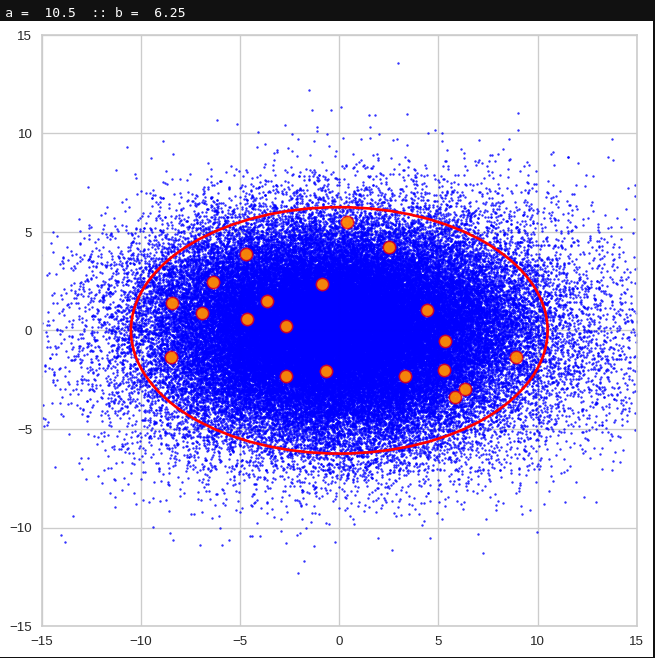For elliptic contour lines see the last post before the present one in this series. The next plot shows the same generated 20 z-vectors for other component-combinations among the first 20 of the most important PCA-components. The plots contain a selection of 60,000 z-points.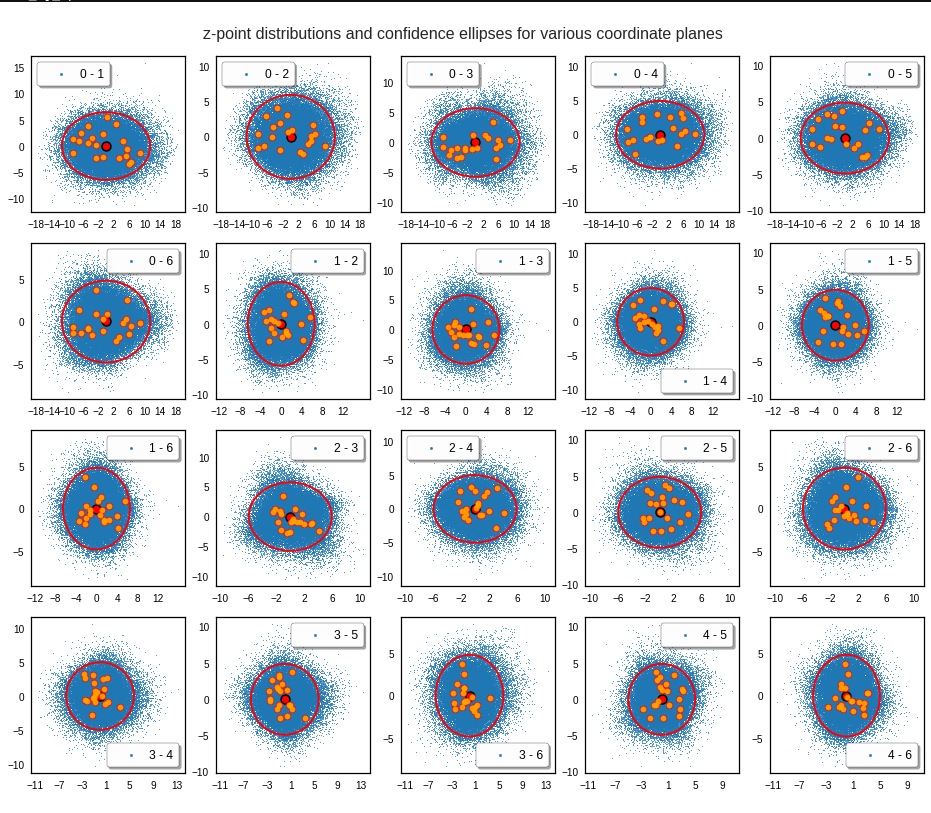The outer z-points points do not always indicate that we have elliptic contours in the denser core of the displayed 2-dimensional distributions. But see the last post for proofs that the inner core inside the red ellipse really displays elliptic contours. You see that all random vectors lie within the 2-σ-ellipses.

The next plot shows the generated z-vectors in the original coordinate system of the latent space. The component values were back-transformed from the PCA-system to the original coordinate system.

Generated statistical z-vectors after an inverse PCA transformation to the original coordinate system of the latent space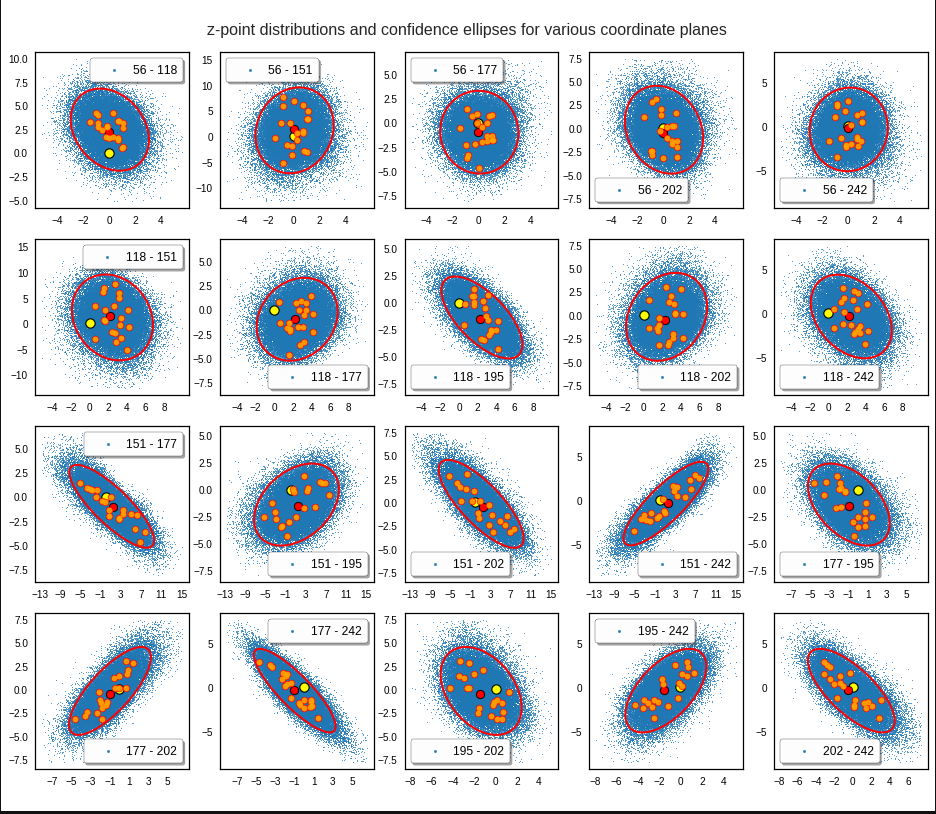We get similar plots for other component pairs. And of course for other generated vectors.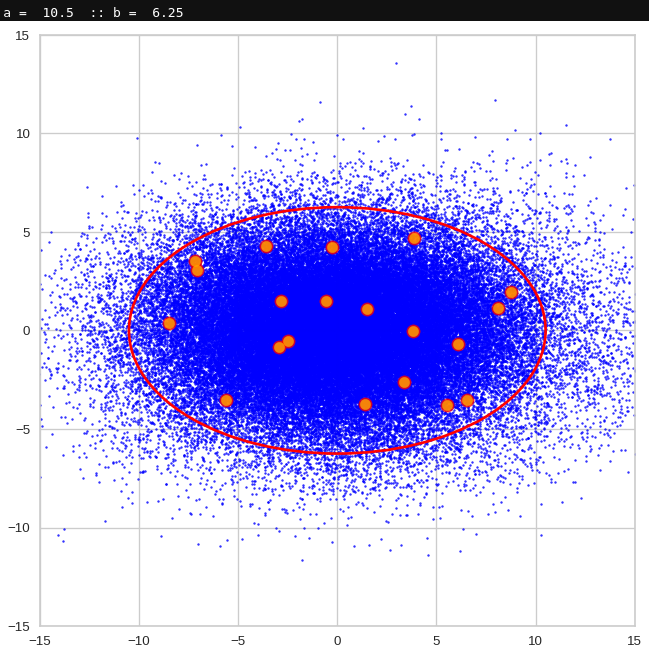Generated statistical z-vectors in the PCA coordinate system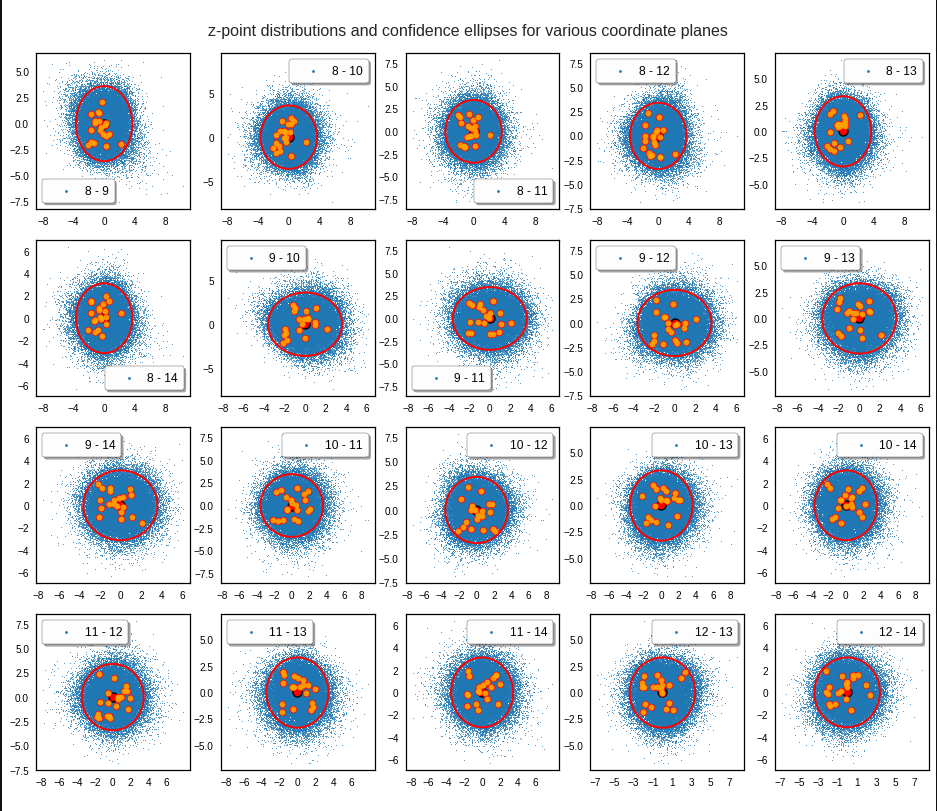Generated statistical z-vectors after an inverse PCA transformation to the original coordinate system of the latent spaceTechnically we have obviously achieved what we wanted: Our generated statistical vectors are distributed within the core of our multidimensional ellipsoid.

Note that this method fortunately works even when we use a limited number of the PCA components, only. This is due to intricate properties of a PCA transformation which guarantee that a back-transformation puts the resulting points close to the original ones even when we omit less important PCA components. I cannot discuss the math-details in this blog. You have to see scientific literature for this. An introduction is e.g. provided by https://arxiv.org/pdf/1404.1100.pdf.

For me this property of the PCA transformation was helpful when I ran into the resolution problem for a proper half-width of the Gaussians. Taking 256 components lead to errors as elliptic conditions for very narrow Gaussians were not properly defined and some of the created vectors left the allowed value ranges.

# Resulting face images

Let us look at some results. First I want to remind you from where we started:

Failed trials with improper random z-vectors based on constant probability densities

A simple random generator used in the beginning was totally inapt to feed the AE’s Decoder with proper statistical z-vectors. And now – look at the following plots. They were produced for a varying number of PCA components between 50 and 120, 100000 statistically selected z-points within a 3 σ-level for the PCA-transformation and various factors 0.6 < fact < 0.8 used upon a half-width corresponding to a confidence level of 2.35 σ: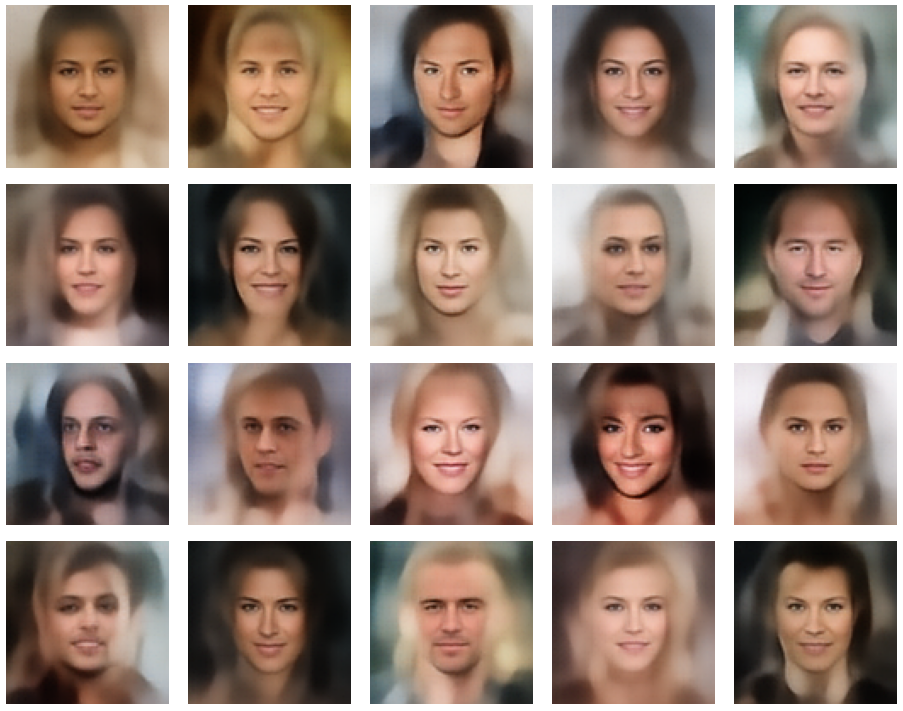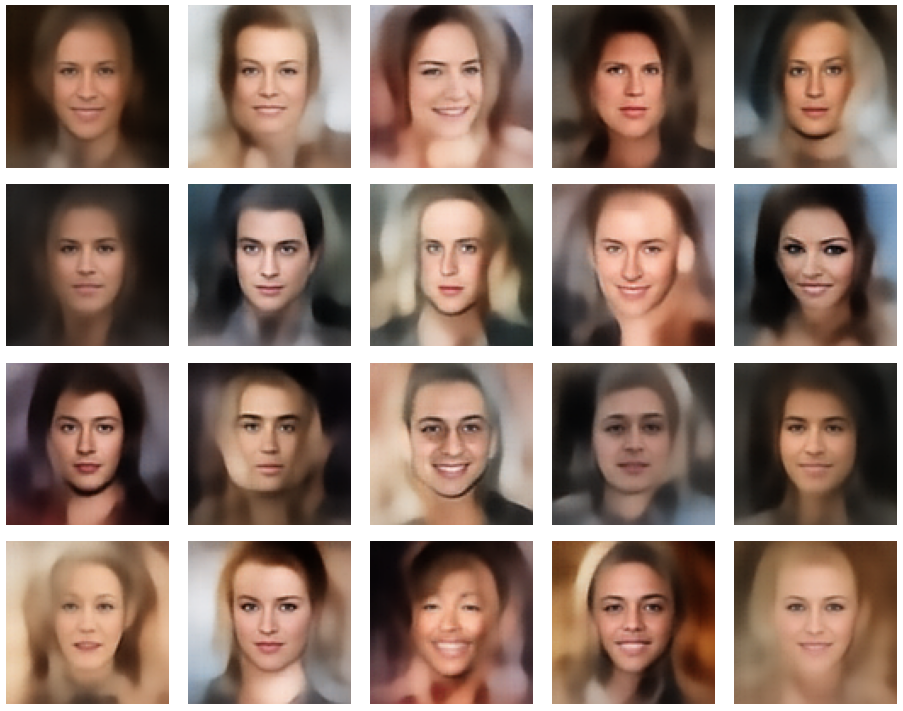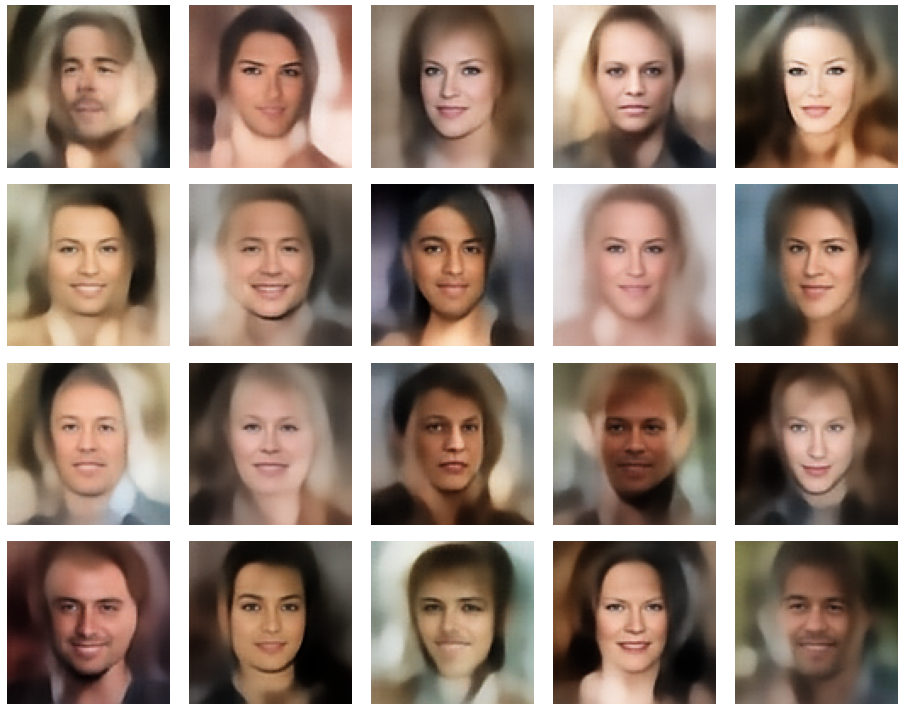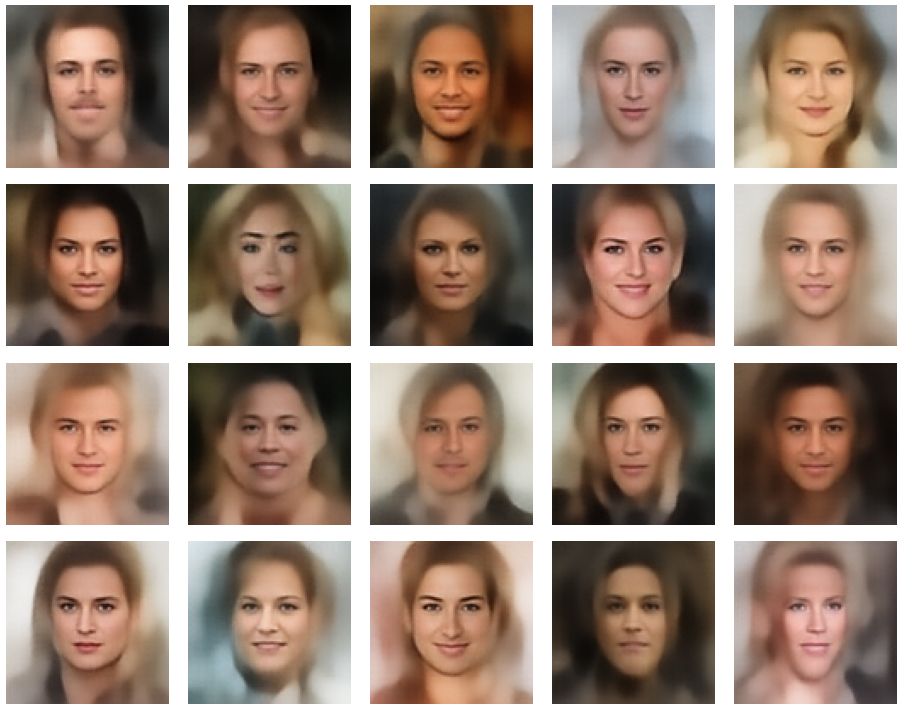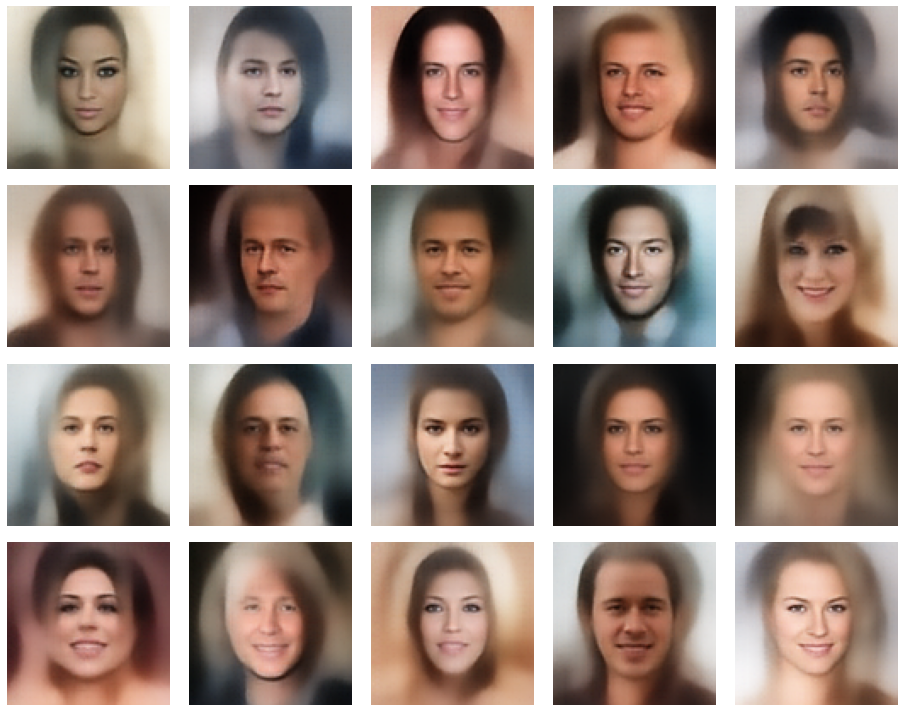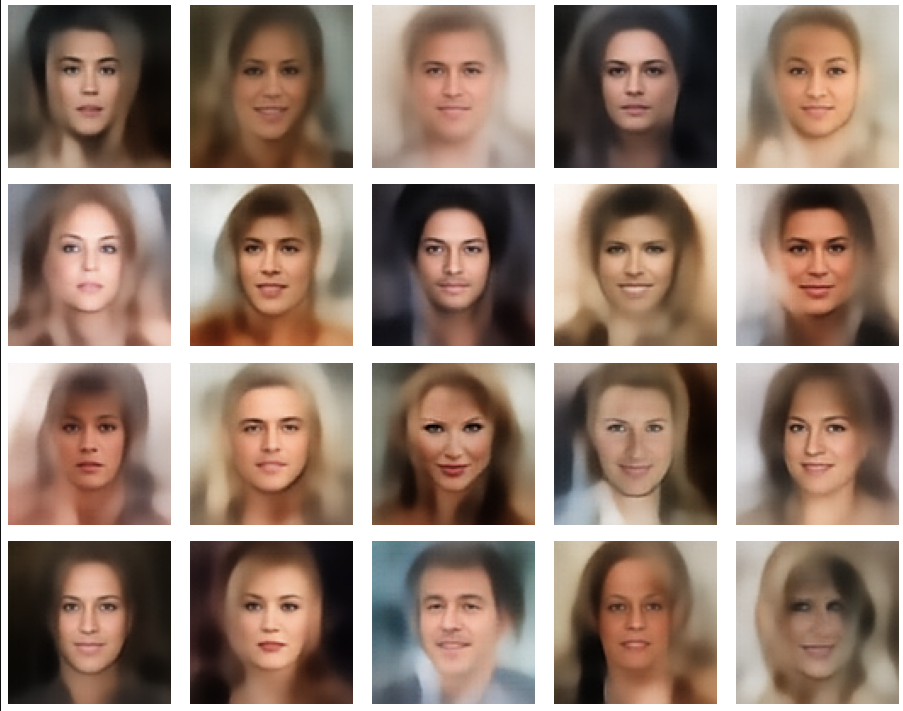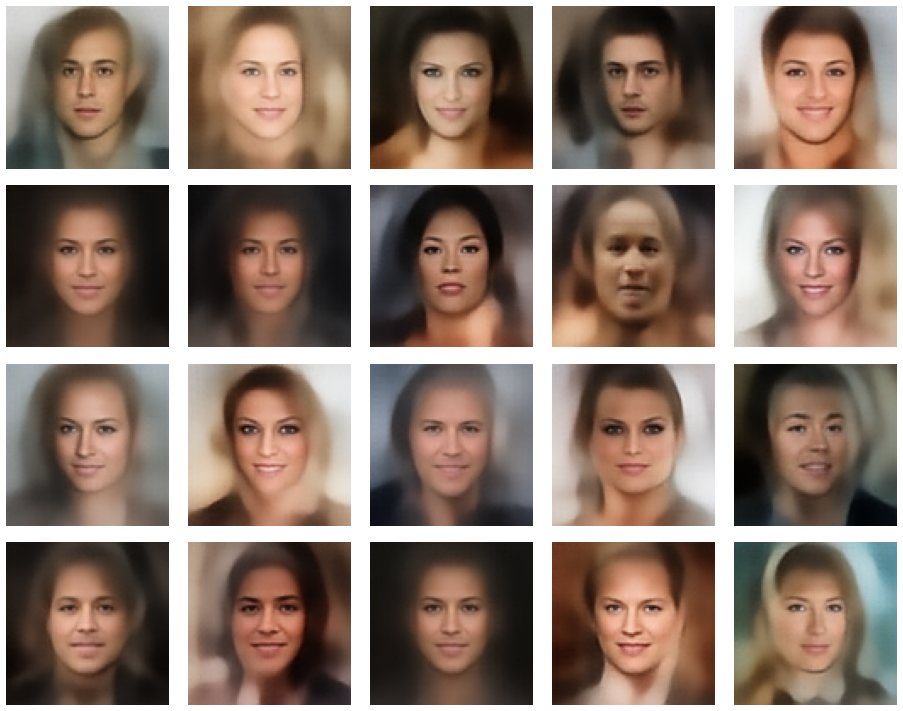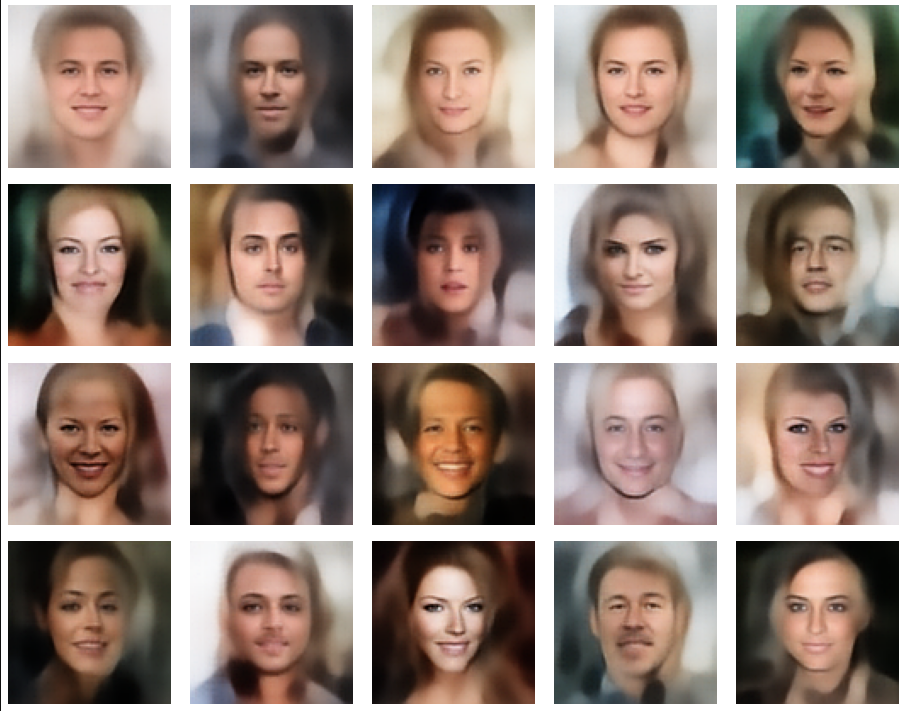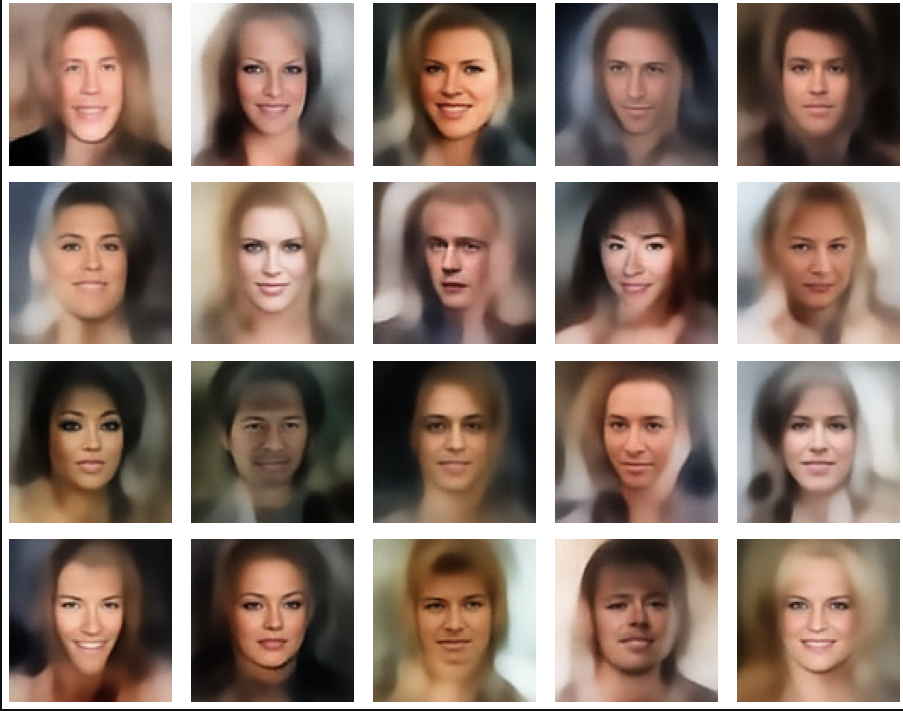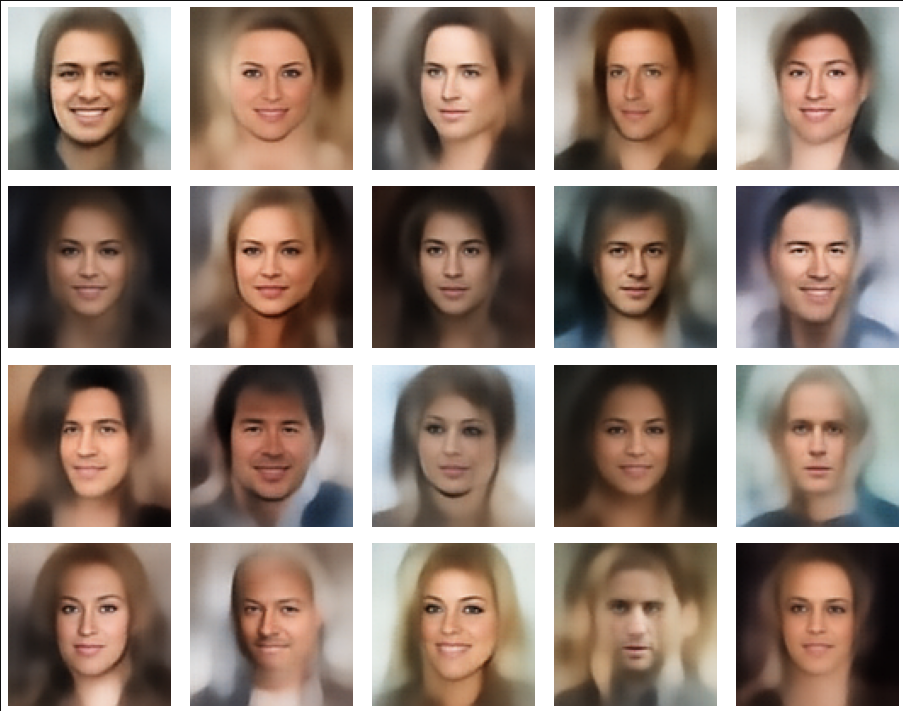In some cases – for a higher number of PCA components – we even see smaller details of the face images and a reasonable transition to some kind of hairdo. Please remember that z_dim = 256 is a pretty low number for the latent space to cover the encoding of face details. And celebrities as covered by CelebA use make-up ….

In case you think the above result is not noteworthy: Please remember that we talk about a simple standard Autoencoder and not about a Variational Autoencoder and neither about a transformer based Autoencoder. No fancy additions to cost functions or special layers. And who ever has read the very instructive book of D. Foster on “Generative Deep Learning” (1st edition, O’Reilly) may compare his images to mine. And I have used a lower resolution of the original images than D. Foster. Just to motivate people to look a bit deeper into properties of data distributions in latent spaces.

# Conclusion and outlook

We have come a lot closer to our objective of using a standard minimal Autoencoder for generative purposes. On our way, we got a much deeper understanding of the vector-distribution a trained AE creates in its latent space for human face images.

The method presented in this post to create reasonable statistical z-vectors still has its limits and there is a lot of open space for improvements. Attentive readers may e.g. ask: Why did he not use confidence ellipses directly? And why not the ellipses found in the original coordinate system of the latent space? And what about micro-correlations? And are there clusters for certain properties as the hair-color, sex, smiling, etc. in the multivariate z-point distribution in the AE’s latent space?

I will discuss these topics in further posts. In the meantime keep in mind that the basic point for turning a standard Autoencoder into a generative tool is to understand how it fills its latent space.

Note also that I myself have speculated in other posts of this blog that failures of using standard AEs for generative purposes may have their ultimate reason in the micro-structure of the z-point distribution. The present results render these previous ideas of mine plain wrong.

# Links to previous posts of this series

Autoencoders and latent space fragmentation – IX – PCA transformation of the z-point distribution for CelebA

Autoencoders and latent space fragmentation – VIII – approximation of the latent vector distribution by a multivariate normal distribution and ellipses

Autoencoders and latent space fragmentation – VII – face images from statistical z-points within the latent space region of CelebA

Autoencoders and latent space fragmentation – VI – image creation from z-points along paths in selected coordinate planes of the latent space

Autoencoders and latent space fragmentation – V – reconstruction of human face images from simple statistical z-point-distributions?

Autoencoders and latent space fragmentation – IV – CelebA and statistical vector distributions in the surroundings of the latent space origin

Autoencoders and latent space fragmentation – III – correlations of latent vector components

Autoencoders and latent space fragmentation – II – number distributions of latent vector components

Autoencoders and latent space fragmentation – I – Encoder, Decoder, latent space

And before we forget it: Besides the Putler in the east there is also an extremist right-wing, semi-fascistic party in Germany on a record high support level in the population of 18%. This is a party which wants to stop all sanctions against the Russian aggressor in the ongoing war in Ukraine. You see the pattern behind this? This party is presently becoming bigger in number of supporters than the government leading social democrats. So, there is more at stake at present in Europe than the war in Ukraine. We need to defend our democracies with all the means of democracies. And its time to ask for more decisive legal action against a party which already is under observation of the German internal secret service.